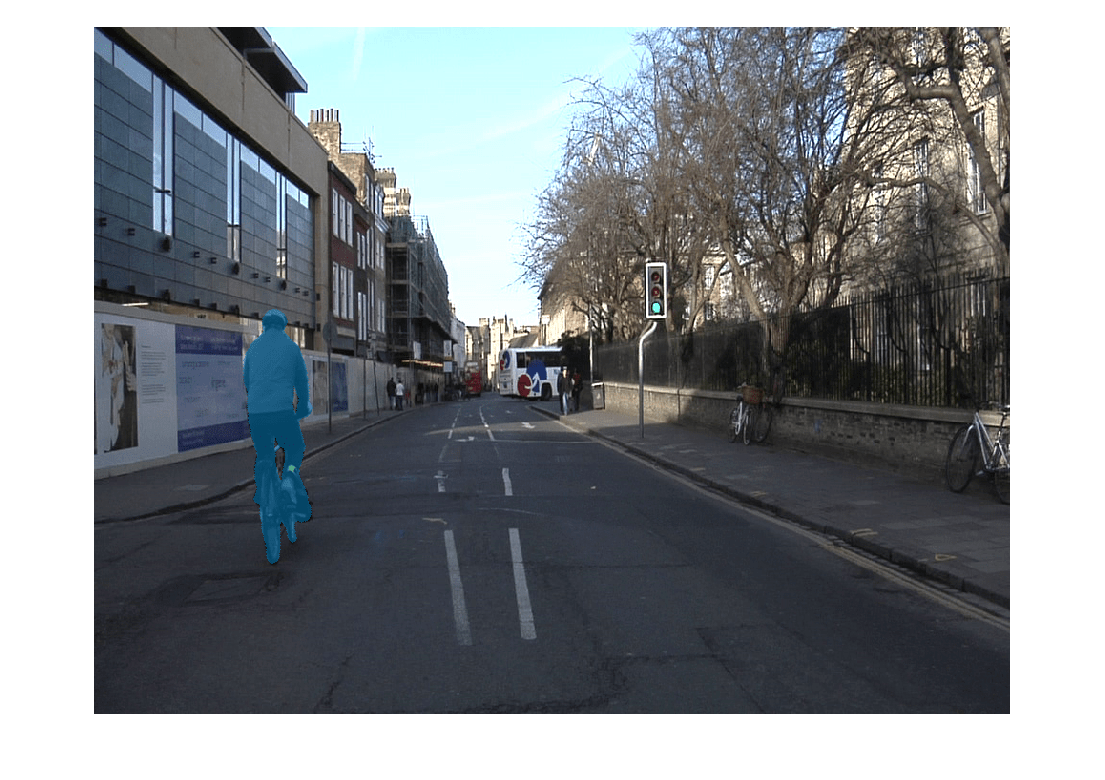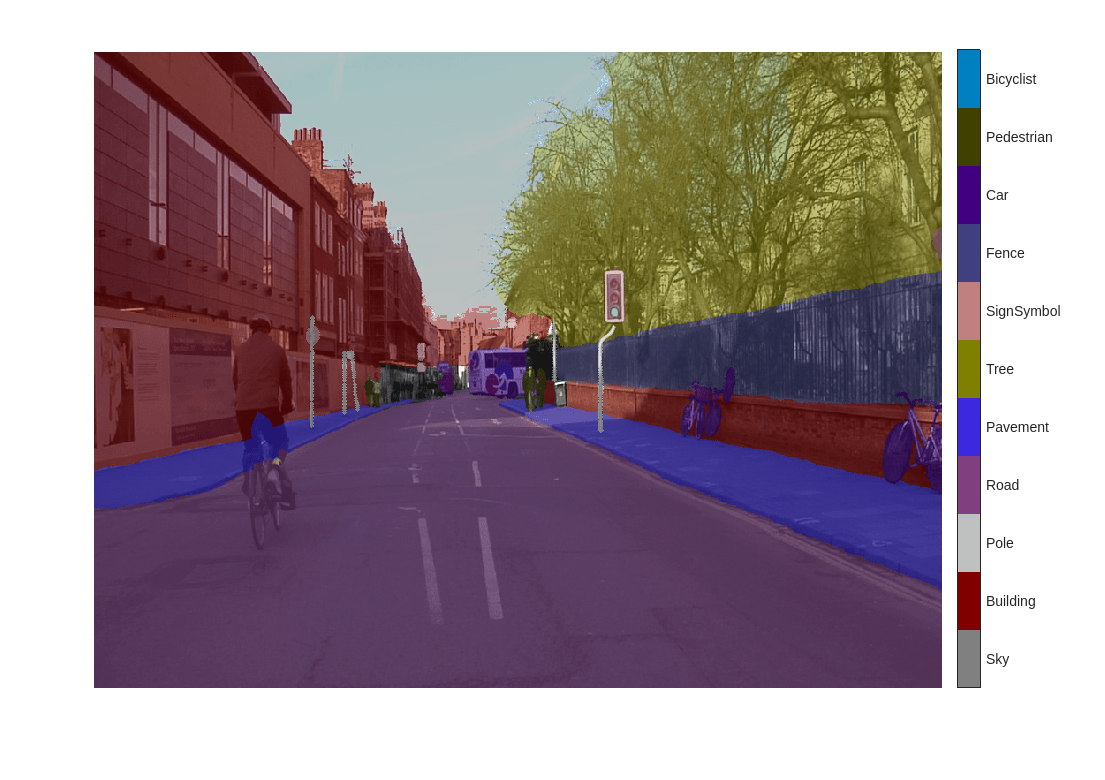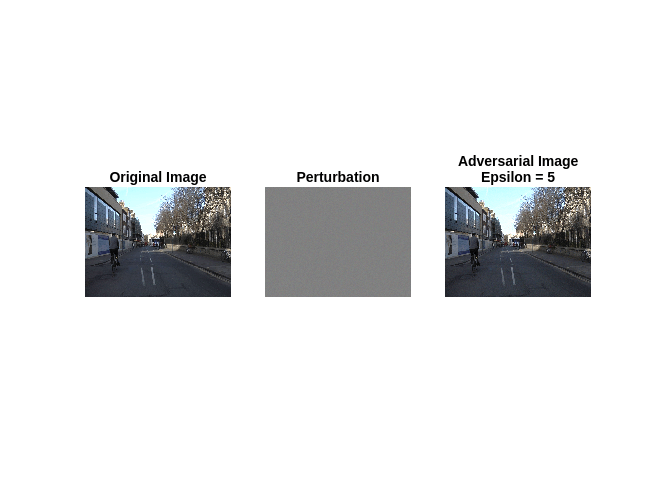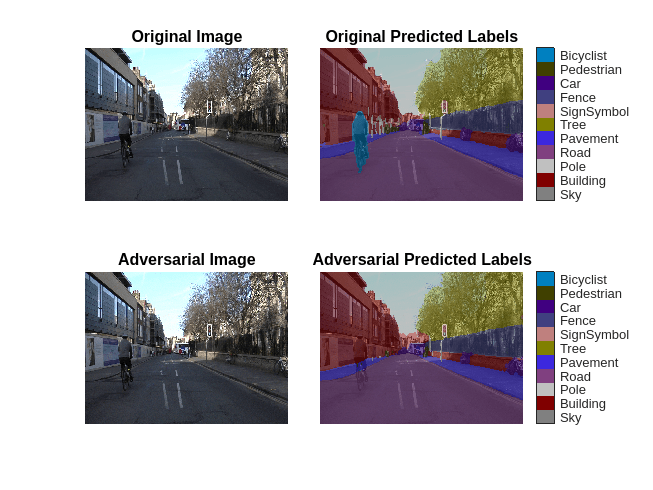# Generate Adversarial Examples for Semantic Segmentation

This example shows how to generate adversarial examples for a semantic segmentation network using the basic iterative method (BIM).

Semantic segmentation is the process of assigning each pixel in an image a class label, for example, car, bike, person, or sky. Applications for semantic segmentation include road segmentation for autonomous driving and cancer cell segmentation for medical diagnosis.

Neural networks can be susceptible to a phenomenon known as adversarial examples , where very small changes to an input can cause it to be misclassified. These changes are often imperceptible to humans. This example shows how to generate an adversarial example for a semantic segmentation network.

This example generates adversarial examples using the CamVid  data set from the University of Cambridge. The CamVid data set is a collection of images containing street-level views obtained while driving. The data set provides pixel-level labels for 32 semantic classes including car, pedestrian, and road.

Load a pretrained semantic segmentation network. This example loads a Deeplab v3+ network trained on the CamVid data set with weights initialized from a pretrained ResNet-18 network. For more information on building and training a Deeplab v3+ semantic segmentation network, see Semantic Segmentation Using Deep Learning.

```pretrainedURL = "https://www.mathworks.com/supportfiles/vision/data/deeplabv3plusResnet18CamVid.mat"; pretrainedFolder = fullfile(tempdir,"pretrainedNetwork"); pretrainedNetwork = fullfile(pretrainedFolder,"deeplabv3plusResnet18CamVid.mat"); if ~exist(pretrainedNetwork,"file") mkdir(pretrainedFolder); disp("Downloading pretrained network (58 MB)..."); websave(pretrainedNetwork,pretrainedURL); end```

Load the network and convert it to a `dlnetwork`.

```data = load(pretrainedNetwork); net = data.net; lgraph = layerGraph(net); lgraph = removeLayers(lgraph,lgraph.Layers(end).Name); net = dlnetwork(lgraph);```

Load an image and its corresponding label image. The image is a street-level view obtained from a car being driven. The label image contains the ground truth pixel labels. In this example, you create an adversarial example that causes the semantic segmentation network to misclassify the pixels in the `Bicyclist` class.

`img = imread("0016E5_08145.png");`

Use the supporting function `convertCamVidLabelImage`, defined at the end of this example, to convert the label image to a categorical array.

`T = convertCamVidLabelImage(imread("0016E5_08145_L.png"));`

The data set contains 32 classes. Use the supporting function `camVidClassNames11`, defined at the end of this example, to reduce the number of classes to 11 by grouping multiple classes from the original data set together.

`classNames = camVidClassNames11;`

Use the supporting function `camVidColorMap11` to create a colormap for the 11 classes.

`cmap = camVidColorMap11;`

Display the image with an overlay showing the pixels with the ground truth label `Bicyclist`.

```classOfInterest = "Bicyclist"; notTheClassOfInterest = T ~= classOfInterest; TClassOfInterest = T; TClassOfInterest(notTheClassOfInterest) = ""; overlayImage = labeloverlay(img,TClassOfInterest,ColorMap=cmap); imshow(overlayImage)```To create an adversarial example, you must specify the adversarial target label for each pixel you want the network to misclassify. In this example, the aim is to get the network to misclassify the `Bicyclist` pixels as another class. Therefore, you need to specify target classes for each of the `Bicyclist` pixels.

Using the supporting function `eraseClass`, defined at the end of this example, create adversarial target labels by replacing all `Bicyclist` pixel labels with the label of the nearest pixel that is not in the `Bicyclist` class .

`TDesired = eraseClass(T,classOfInterest);`

```overlayImage = labeloverlay(img,TDesired,ColorMap=cmap); figure imshow(overlayImage) pixelLabelColorbar(cmap,classNames);```The labels of the `Bicyclist` pixels are now `Road`, `Building`, or `Pavement`.

#### Prepare Data

To create the adversarial example using the image and the adversarial target labels, you must first prepare the image and the labels.

Prepare the image by converting it to a `dlarray`.

`X = dlarray(single(img), "SSCB");`

Prepare the label by one-hot encoding it. Because some of the pixels have undefined labels, replace `NaN` values with `0`.

```TDesired = onehotencode(TDesired,3,"single",ClassNames=classNames); TDesired(isnan(TDesired)) = 0; TDesired = dlarray(TDesired,"SSCB");```

Use the adversarial target labels to create an adversarial example using the basic iterative method (BIM) . The BIM iteratively calculates the gradient ${\nabla }_{X}L\left(X,T\right)$ of the loss function $L$ with respect to the image $X$ you want to find an adversarial example for and the adversarial target labels $T$. The negative of this gradient describes the direction to "push" the image in to make the output closer to the desired class labels.

The adversarial example image is calculated iteratively as follows:

${\mathit{X}}_{\mathrm{adv}}=\mathit{X}-\alpha .\mathrm{sign}\left({\nabla }_{\mathit{X}}\mathit{L}\left(\mathit{X},\mathit{T}\right)\right)$.

Parameter $\alpha$ controls the size of the push for a single iteration. After each iteration, clip the perturbation to ensure the magnitude does not exceed $ϵ$. Parameter $ϵ$ defines a ceiling on how large the total change can be over all the iterations. A larger $ϵ$ value increases the chance of generating a misclassified image, but makes the change in the image more visible.

Set the `epsilon` value to `5`, set the step size `alpha` to `1`, and perform `10` iterations.

```epsilon = 5; alpha = 1; numIterations = 10;```

Keep track of the perturbation and clip any values that exceed `epsilon`.

```delta = zeros(size(X),like=X); for i = 1:numIterations gradient = dlfeval(@targetedGradients,net,X+delta,TDesired); delta = delta - alpha*sign(gradient); delta(delta > epsilon) = epsilon; delta(delta < -epsilon) = -epsilon; end XAdvTarget = X + delta;```

Display the original image, the perturbation added to the image, and the adversarial image.

`showAdversarialImage(X,XAdvTarget,epsilon)`The added perturbation is imperceptible, demonstrating how adversarial examples can exploit robustness issues within a network.

### Predict Pixel Labels

Predict the class labels of the original image and the adversarial image using the semantic segmentation network.

```Y = semanticseg(extractdata(X),net); YAdv = semanticseg(extractdata(XAdvTarget),net);```

Display an overlay of the predictions for both images.

```overlayImage = labeloverlay(uint8(extractdata(X)),Y,ColorMap=cmap); overlayAdvImage = labeloverlay(uint8(extractdata(XAdvTarget)),YAdv,ColorMap=cmap); figure tiledlayout("flow",TileSpacing="tight") nexttile imshow(uint8(extractdata(X))) title("Original Image") nexttile imshow(overlayImage) pixelLabelColorbar(cmap,classNames); title("Original Predicted Labels") nexttile imshow(uint8(extractdata(XAdvTarget))) title("Adversarial Image") nexttile imshow(overlayAdvImage) pixelLabelColorbar(cmap,classNames); title("Adversarial Predicted Labels")```The network correctly identifies the bicyclist in the original image. However, because of imperceptible perturbation, the network mislabels the bicyclist in the adversarial image.

### Supporting Functions

#### Convert CamVid Label Image to a Categorical

The supporting function `convertCamVidLabelImage` takes as input a label image from the CamVid data set and converts it to a categorical array.

```function labelImage = convertCamVidLabelImage(image) colorMap32 = camVidColorMap32; map32To11 = cellfun(@(x,y)repmat(x,size(y,1),1), ... num2cell((1:numel(colorMap32))'), ... colorMap32, ... UniformOutput=false); colorMap32 = cat(1,colorMap32{:}); map32To11 = cat(1,map32To11{:}); labelImage = rgb2ind(double(image)./255,colorMap32); labelImage = map32To11(labelImage+1); labelImage = categorical(labelImage,1:11,camVidClassNames11); end```

#### CamVid Color Map (32 classes)

The supporting function `camVidColorMap32` returns the color map for the 32 original classes in the CamVid data set.

```function cmap = camVidColorMap32 cmap = { % Sky [ 128 128 128 ] % Building [ 0 128 64 % Bridge 128 0 0 % Building 64 192 0 % Wall 64 0 64 % Tunnel 192 0 128 % Archway ] % Pole [ 192 192 128 % Column_Pole 0 0 64 % TrafficCone ] % Road [ 128 64 128 % Road 128 0 192 % LaneMkgsDriv 192 0 64 % LaneMkgsNonDriv ] % Pavement [ 0 0 192 % Sidewalk 64 192 128 % ParkingBlock 128 128 192 % RoadShoulder ] % Tree [ 128 128 0 % Tree 192 192 0 % VegetationMisc ] % SignSymbol [ 192 128 128 % SignSymbol 128 128 64 % Misc_Text 0 64 64 % TrafficLight ] % Fence [ 64 64 128 % Fence ] % Car [ 64 0 128 % Car 64 128 192 % SUVPickupTruck 192 128 192 % Truck_Bus 192 64 128 % Train 128 64 64 % OtherMoving ] % Pedestrian [ 64 64 0 % Pedestrian 192 128 64 % Child 64 0 192 % CartLuggagePram 64 128 64 % Animal ] % Bicyclist [ 0 128 192 % Bicyclist 192 0 192 % MotorcycleScooter ] % Void [ 0 0 0 % Void ] }; % Normalize between [0 1]. cmap = cellfun(@(x)x./255,cmap,UniformOutput=false); end```

#### CamVid Color Map (11 classes)

The supporting function `camVidColorMap11` returns the color map for the 11 umbrella classes in the CamVid data set.

```function cmap = camVidColorMap11 cmap = [ 128 128 128 % Sky 128 0 0 % Building 192 192 192 % Pole 128 64 128 % Road 60 40 222 % Pavement 128 128 0 % Tree 192 128 128 % SignSymbol 64 64 128 % Fence 64 0 128 % Car 64 64 0 % Pedestrian 0 128 192 % Bicyclist ]; % Normalize between [0 1]. cmap = cmap ./ 255; end```

#### CamVid Labels (11 classes)

The supporting function `classNames` returns the 11 umbrella classes of the CamVid data set.

```function classNames = camVidClassNames11 classNames = [ "Sky" "Building" "Pole" "Road" "Pavement" "Tree" "SignSymbol" "Fence" "Car" "Pedestrian" "Bicyclist" ]; end```

#### Pixel Label Colorbar Function

The supporting function `pixelLabelColorbar` adds a colorbar to the current axis. The colorbar is formatted to display the class names with the color.

```function pixelLabelColorbar(cmap, classNames) % Add a colorbar to the current axis. The colorbar is formatted % to display the class names with the color. colormap(gca,cmap) % Add colorbar to current figure. c = colorbar("peer", gca); % Use class names for tick marks. c.TickLabels = classNames; numClasses = size(cmap,1); % Center tick labels. c.Ticks = 1/(numClasses*2):1/numClasses:1; % Remove tick mark. c.TickLength = 0; end```

#### Erase Class Function

The supporting function `eraseClass` removes class `classToErase` from the label image `T` by relabeling the pixels in class `classToErase`. For each pixel in class `classToErase`, the `eraseClass` function sets the pixel label to the class of the nearest pixel not in class `classToErase`.

```function TDesired = eraseClass(T,classToErase) classToEraseMask = T == classToErase; [~,idx] = bwdist(~(classToEraseMask | isundefined(T))); TDesired = T; TDesired(classToEraseMask) = T(idx(classToEraseMask)); end```

```function gradient = targetedGradients(net,X,target) Y = predict(net,X); loss = mse(Y,target); gradient = dlgradient(loss,X); end```

Show an image, the corresponding adversarial image, and the difference between the two (perturbation).

```function showAdversarialImage(image,imageAdv,epsilon) figure tiledlayout(1,3,TileSpacing="compact") nexttile imgTrue = uint8(extractdata(image)); imshow(imgTrue) title("Original Image") nexttile perturbation = uint8(extractdata(imageAdv-image+127.5)); imshow(perturbation) title("Perturbation") nexttile advImg = uint8(extractdata(imageAdv)); imshow(advImg) title("Adversarial Image" + newline + "Epsilon = " + string(epsilon)) end```

### References

 Goodfellow, Ian J., Jonathon Shlens, and Christian Szegedy. “Explaining and Harnessing Adversarial Examples.” Preprint, submitted March 20, 2015. https://arxiv.org/abs/1412.6572.

 Brostow, Gabriel J., Julien Fauqueur, and Roberto Cipolla. “Semantic Object Classes in Video: A High-Definition Ground Truth Database.” Pattern Recognition Letters 30, no. 2 (January 2009): 88–97. https://doi.org/10.1016/j.patrec.2008.04.005.

 Fischer, Volker, Mummadi Chaithanya Kumar, Jan Hendrik Metzen, and Thomas Brox. “Adversarial Examples for Semantic Image Segmentation.” Preprint, submitted March 3, 2017. http://arxiv.org/abs/1703.01101.

 Kurakin, Alexey, Ian Goodfellow, and Samy Bengio. “Adversarial Examples in the Physical World.” Preprint, submitted February 10, 2017. https://arxiv.org/abs/1607.02533.